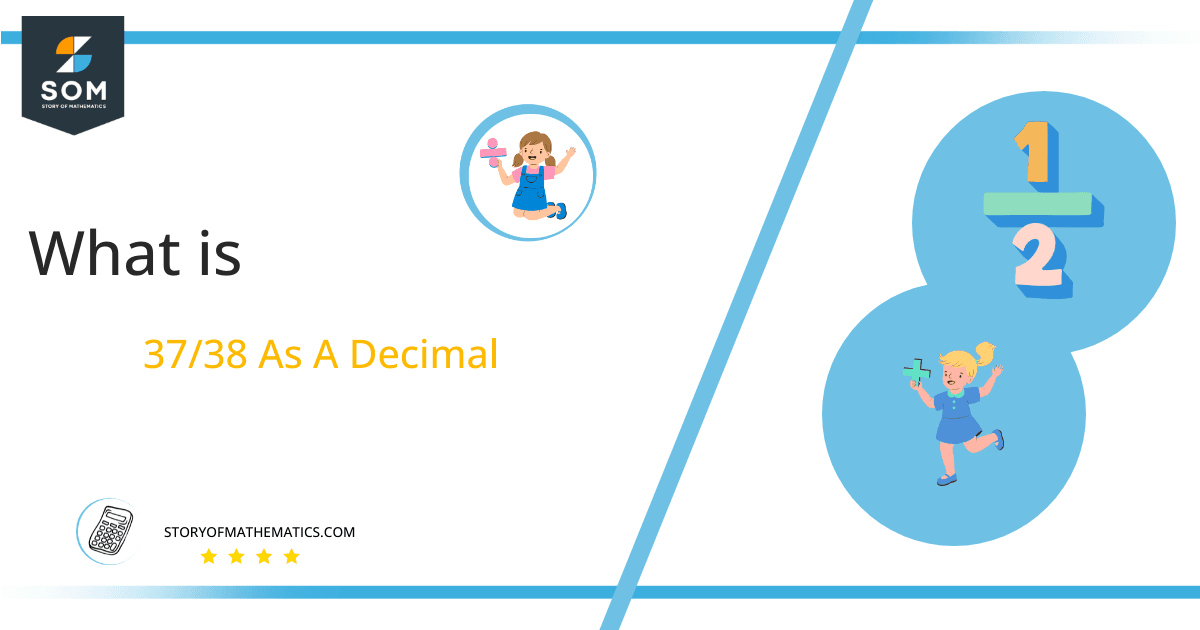# What Is 37/38 as a Decimal + Solution With Free Steps

The fraction 37/38 as a decimal is equal to 0.973.

The decimal form of a fraction is obtained using the long division process. The decimals are numerical values obtained as a quotient when a long division is performed. The current fraction upon solving produces a recurring decimal with ‘9‘ and ‘6‘ as its two repeating digits.

Here, we are more interested in the division types that result in a Decimal value, as this can be expressed as a Fraction. We see fractions as a way of showing two numbers having the operation of Division between them that result in a value that lies between two Integers.Now, we introduce the method used to solve said fraction to decimal conversion, called Long Division, which we will discuss in detail moving forward. So, let’s go through the Solution of fraction 37/38.

## Solution

First, we convert the fraction components, i.e., the numerator and the denominator, and transform them into the division constituents, i.e., the Dividend and the Divisor, respectively.

This can be done as follows:

Dividend = 37

Divisor = 38

Now, we introduce the most important quantity in our division process: the Quotient. The value represents the Solution to our division and can be expressed as having the following relationship with the Division constituents:

Quotient = Dividend $\div$ Divisor = 37 $\div$ 38

This is when we go through the Long Division solution to our problem. The long division for fraction 37/38 can be seen in figure 1 below.Figure 1

## 37/38 Long Division Method

We start solving a problem using the Long Division Method by first taking apart the division’s components and comparing them. As we have 37 and 38, we can see how 37 is Smaller than 38, and to solve this division, we require that 37 be Bigger than 38.

This is done by multiplying the dividend by 10 and checking whether it is bigger than the divisor or not. If so, we calculate the Multiple of the divisor closest to the dividend and subtract it from the Dividend. This produces the Remainder, which we then use as the dividend later.

Now, we begin solving for our dividend 37, which after getting multiplied by 10 becomes 370.

We take this 370 and divide it by 38; this can be done as follows:

370 $\div$ 38 $\approx$ 9

Where:

38 x 9 = 342

This will lead to the generation of a Remainder equal to 370 – 342 = 28. Now this means we have to repeat the process by Converting the 28 into 280 and solving for that:

280 $\div$ 38 $\approx$ 7

Where:

38 x 7 = 266

This, therefore, produces another Remainder which is equal to 280 – 266 = 14. Now we must solve this problem to Third Decimal Place for accuracy, so we repeat the process with dividend 140.

140 $\div$ 38 $\approx$ 3

Where:

38 x 3 = 114

Finally, we have a Quotient generated after combining the three pieces of it as 0.973, with a Remainder equal to 26.Images/mathematical drawings are created with GeoGebra.